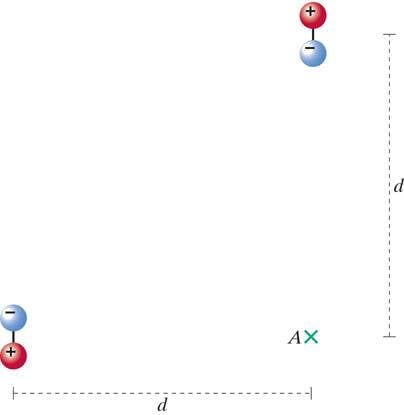# Electric Dipole Moment and electric field

## Homework Statement

Two dipoles are oriented as shown in the diagram below. Each dipole consists of two charges +q and -q, held apart by a rod of length s, and the center of each dipole is a distance d from location A. If q = 6 nC, s = 1 mm, and d = 6 cm, what is the electric field at location A?## Homework Equations

E(axis) = qk x (r-s/2)^2 - (r+s/2)^2)^-1

E(axis) = 2qks/d^3 when d>>s

k=8.99E9 N^2 m / C^2

## The Attempt at a Solution

Since d>>s, the x-axis dipole's angle can be considered linear, and thus, the E-field for the x component is 0.

For the y-axis dipole, d>>s allows us to use the second equation, E(axis) = 2qks/d^3.

E = 2 (8.99E9) (6E-9) (0.001) / (0.06^3) = 500.

My answer would be <0,500,0>. I got the x and z components correct, but the y component is not. Why is that? Did I get the x component right for the wrong reasons or something?

ehild
Homework Helper
The y component of the electric field arising from the x-axis dipole is not 0.

ehild

Oh, whoops. You're right.

With the Y component for the x-axis, it would be E(y) = kqs/y^3 = (8.99E9 * 6E-9 * 0.001) / (0.06^3) = 250.

250 + 500 = 750; <0,750,0>

Thanks.